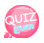# creating function

In this tutorial we will create a function.
In JavaScript we can go back and forth with functions that we already have, but we can create our own.
Also, since we are building a function, we can name the function whatever we want.

### how to create function

First write down function. Then name the function, open and close parentheses, open and close square brackets, and end !!

```function functionName(){
}```

Same as above. Then write the statement to execute in {}.
And a function works only when the function is called. In other words, if you write it like that, it won't work.

### how to call function

How to call a function is the end of function name()!

In other words,

`functionName();`

So let's create a function that prints the hello world and call it.

```function hello(){
document.write("Hello World!!");
}```

Create a function called hello and put the Hello World output in {}.
And the function call

`hello();`

If you write as above, it is called.
Let's see through an example.
It is a source without function call statement.

```<!DOCTYPE html>
<html>
<meta charset="utf-8" />
<title>JavaScript</title>
<script>
function hello(){
document.write("Hello World!!");
}
</script>
<body>
</body>
</html>```

If you run this source by clicking the View on Web button, you will see that nothing is output.
Now let's test it by putting a function call statement.

```<!DOCTYPE html>
<html>
<meta charset="utf-8" />
<title>JavaScript</title>
<script>
function hello(){
document.write("Hello World!!");
}
hello();
</script>
<body>
</body>
</html>```

### If you run the example above, you will see the output hello world.

You can put a formula inside a function or write the statement you need. ^^
Now let's create a function that does 5 + 3.

```<!DOCTYPE html>
<html>
<meta charset="utf-8" />
<title>JavaScript</title>
<script>
}
</script>
<body>
</body>
</html>```

Now let's create a function that does 24 + 10.

```<!DOCTYPE html>
<html>
<meta charset="utf-8" />
<title>JavaScript</title>
<script>
}

</script>
<body>
</body>
</html>```

I made a function that calculates 5 + 3 as above, and I made a function that does 24 + 10.
This addition function does not mean that if you add another function or make another function, you do not create a function.
To illustrate this, I created an example source twice with the same function.
Then what should you use if the input functions are different with the same function as above? When you call a function right away, there is a way to send it with a value.
In other words, if you do 24 + 10, add function can be assigned to function and 24 and 10 can be sent at function call.
That way, when you call the function, you put the value in parentheses like this:

If you call the function as above, 24 and 10 will be transferred to add function when the function is called, and the sum of 24 and 10 will be calculated by the formula and the value calculated by the output statement will be output.
If you send a value when you call the function like above, what should you do when creating the function?
Put as many variables in the parentheses of the function as you send. So 24 is put in the variable a and 10 is put in the variable b.

### JavaScript

```function add(a,b){
}```

You can declare it as above. A and b above are called parameters (parameters).
Then 24 is the value of a and 10 is the value of b.
Let's see through the actual example.

```<!DOCTYPE html>
<html>
<meta charset="utf-8" />
<title>JavaScript</title>
<script>
}
</script>
<body>
</body>
</html>```

If you do a large job later, the source is more recyclable and can be used conveniently. ^^ It can be a string in addition to numbers, so please test it.

```function string_output(str){
document.write(str);
}
string_output("Enter the function and I'll print it");```

In the example source,

```<!DOCTYPE html>
<html>
<meta charset="utf-8" />
<title>JavaScript</title>
<script>
function string_output(str){
document.write(str);
}
string_output("Enter the function and I'll print it");
</script>